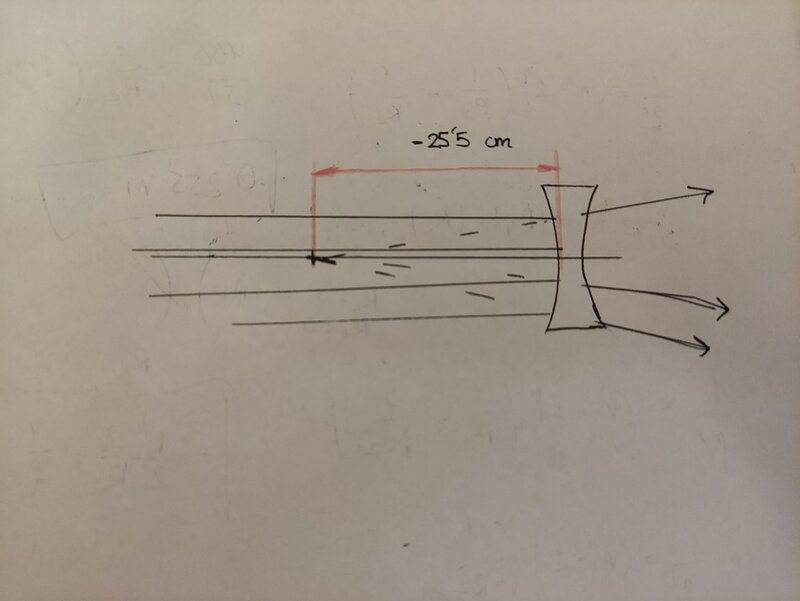# Geometrical optics problem

Marioweee
Homework Statement:
We have a divergent lens on which a beam of parallel rays strikes from left to right. Part of the light reflects off the first face and the reflected rays converge at the point indicated by the arrow, which is - 25.5 cm from the vertex of the first lens surface. Assuming that the lens is symmetrical, that is, that "R1= -R2 &, which is thin, and whose refractive index is 1.5, state the value of the focal point of the lens with its sign.
Relevant Equations:
---
I have recently started with geometric optics and I do not quite understand what this problem asks of me. According to the statement, the focal point of the lens would be -25.5cm, right? That is, it is only a problem of concepts where it is not necessary to take into account the radii of the lenses or the refractive index, right?
Maybe I am very wrong that is why I ask for some help.
This is the picture of the problem that I have done.Mentor
According to the statement, the focal point of the lens would be -25.5cm, right?
Why would you think that? Hint: Solve the problem in two steps. Step one: Treat the first surface of the lens as a mirror. What can you then deduce?

Marioweee
If the first surface is treated as a spherical mirror then f=R/2. From this equation we can determine the value of R1. Then, from the Lensmarker's equation I could determine the focal length of the system, right?

Mentor
If the first surface is treated as a spherical mirror then f=R/2. From this equation we can determine the value of R1. Then, from the Lensmarker's equation I could determine the focal length of the system, right?
Right!

Marioweee
Thank you very much for your help